# Selina Solutions Concise Maths Class 10 Chapter 20 Cylinder, Cone and Sphere (Surface Area and Volume) Exercise 20(G)

Mathematics is one of the most scoring subjects in ICSE Class 10. Hence, having a strong grip over the concepts is vital for students to secure high marks in their board exams. That is why BYJU’S presents you the Selina Solutions for Class 10 Maths to meet the needs of all students. The solutions are in accordance with the latest ICSE patterns. Further, the solutions to the Concise Selina Solutions for Class 10 Maths Chapter 20 Cylinder, Cone and Sphere (Surface Area and Volume) Exercise 20(G) can be accessed in PDF format, from the link given below.

## Selina Solutions Concise Maths Class 10 Chapter 20 Cylinder, Cone and Sphere (Surface Area and Volume) Exercise 20(G) Download PDF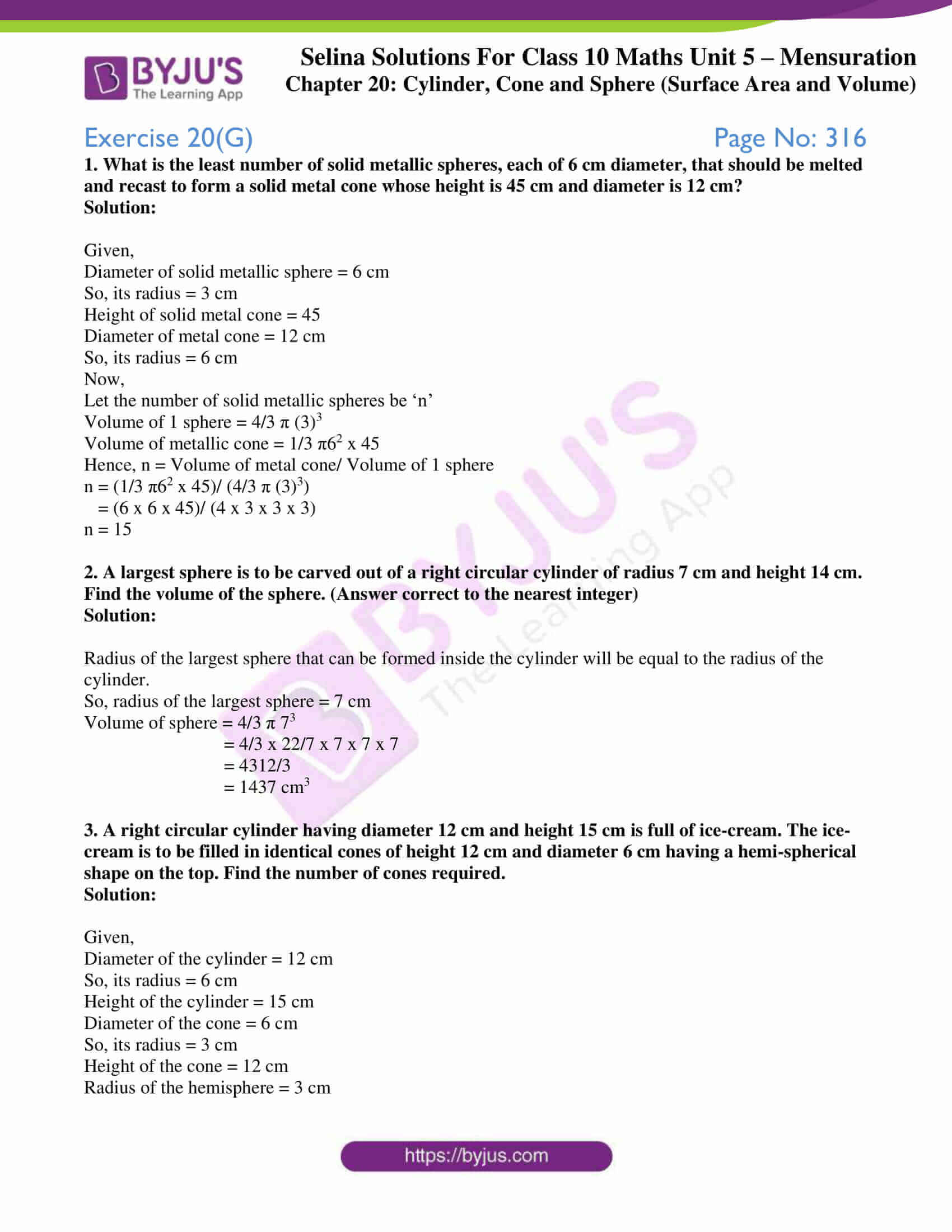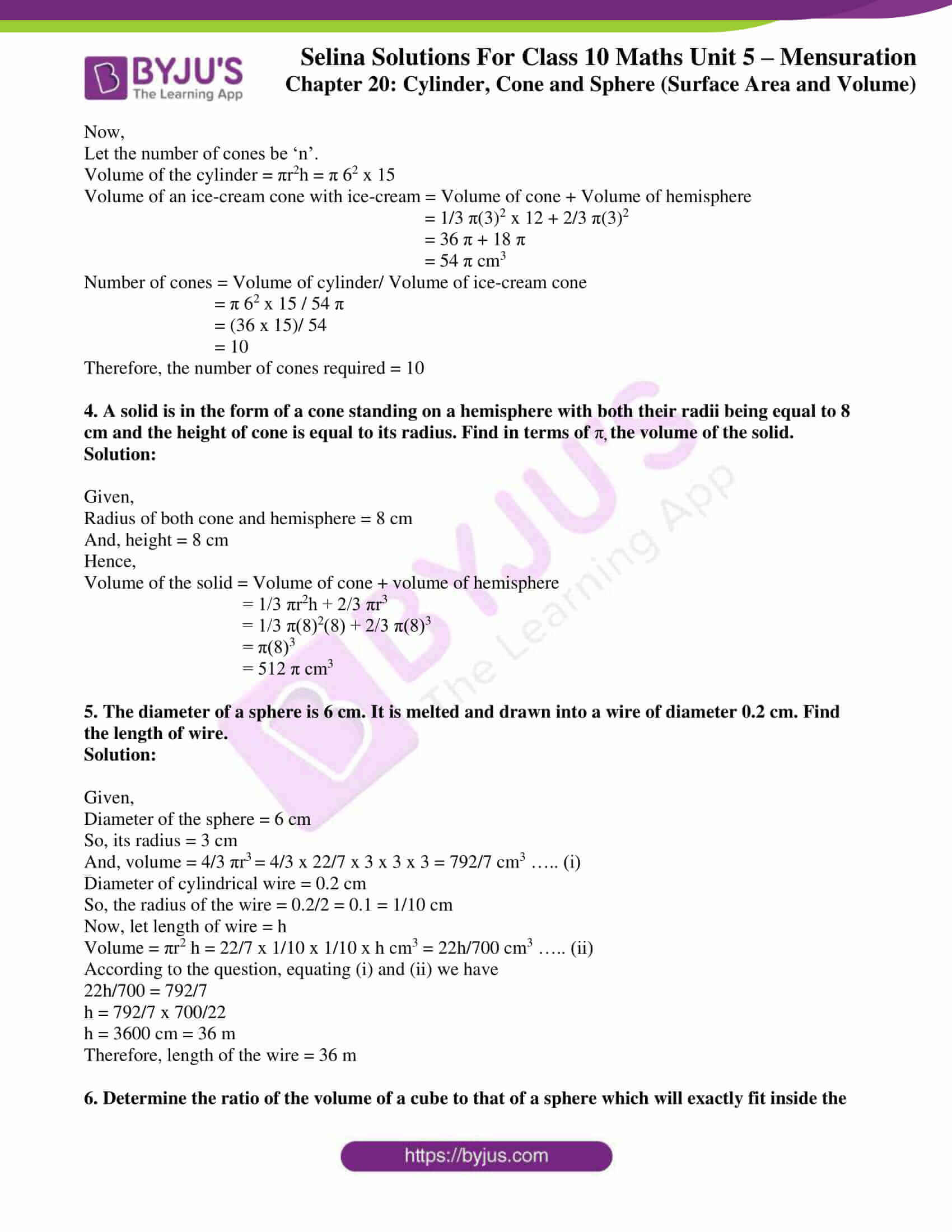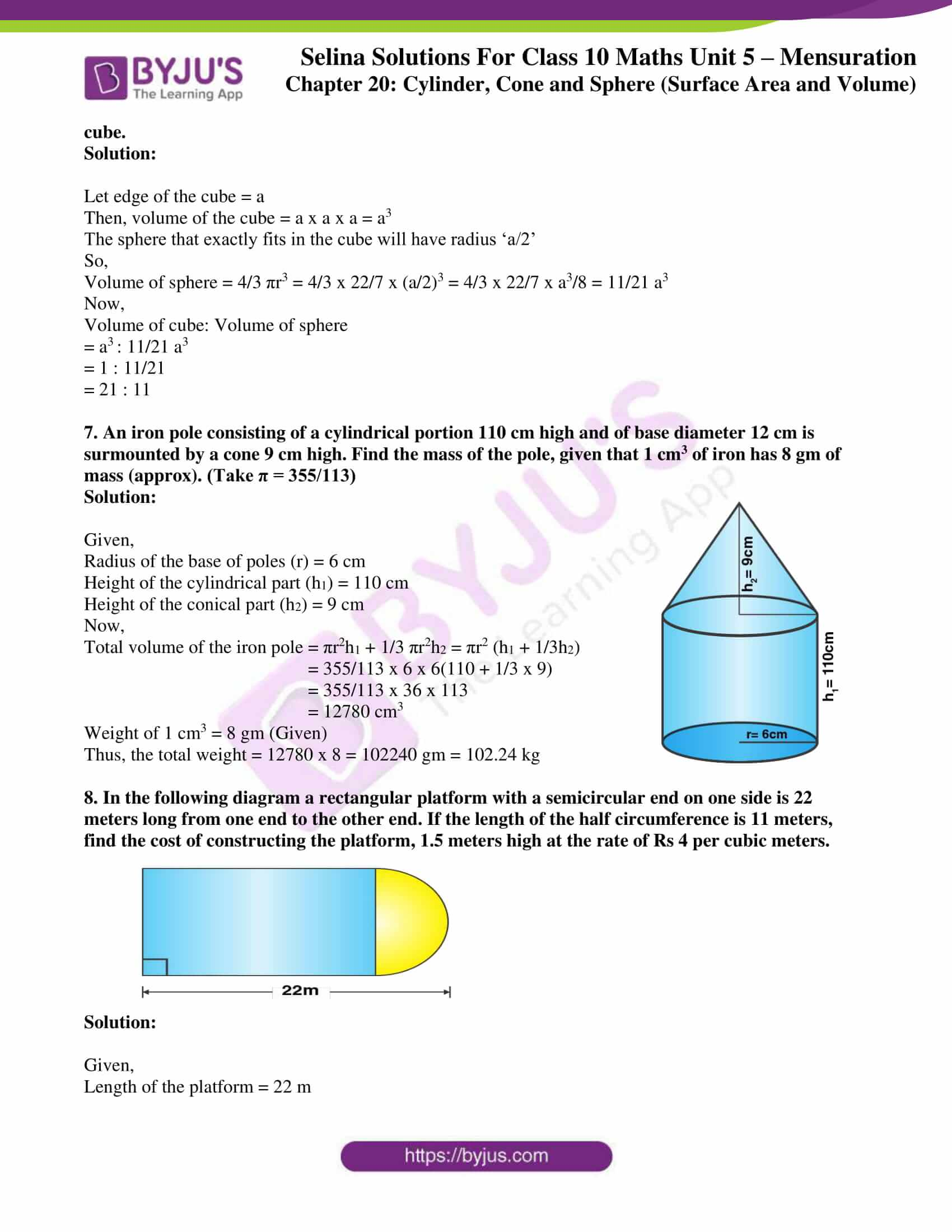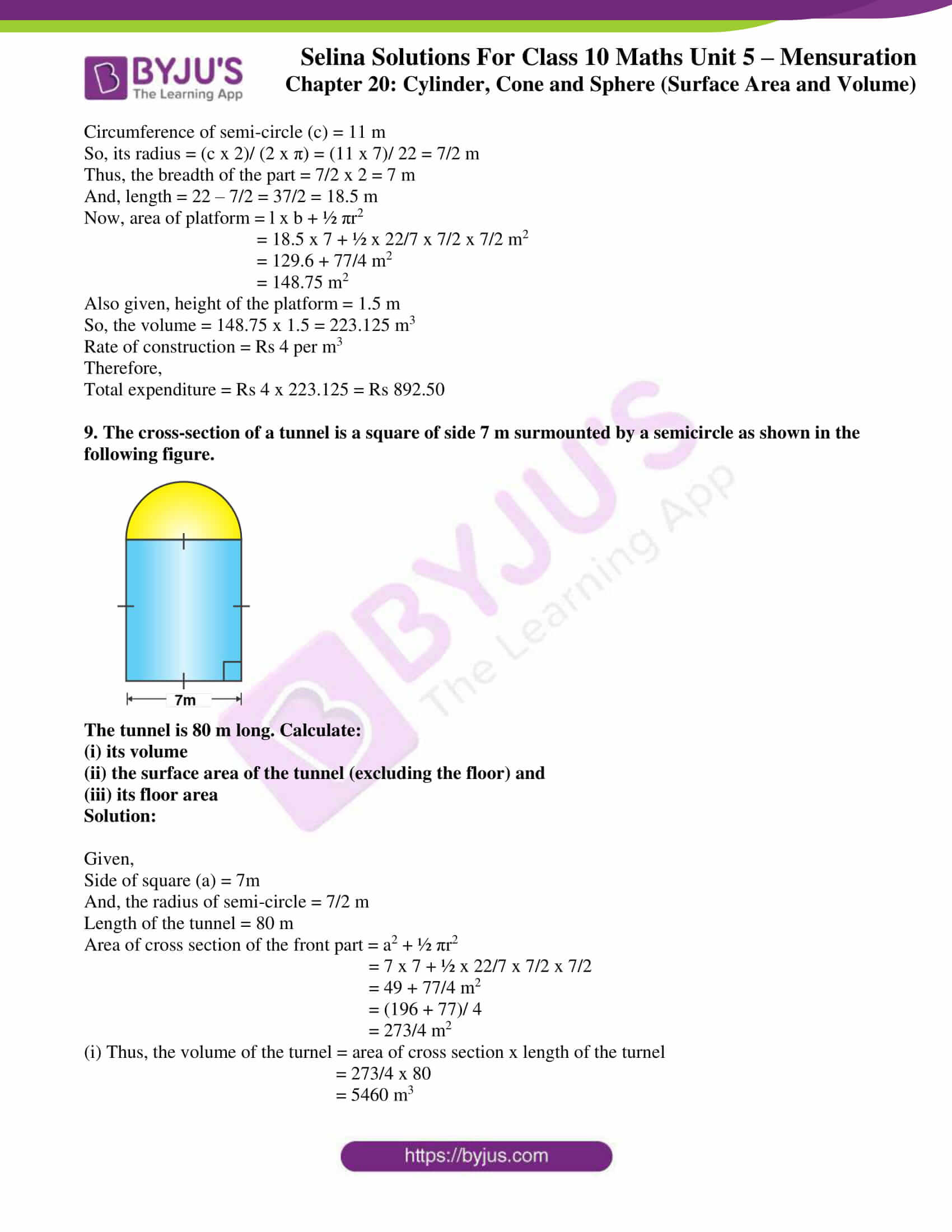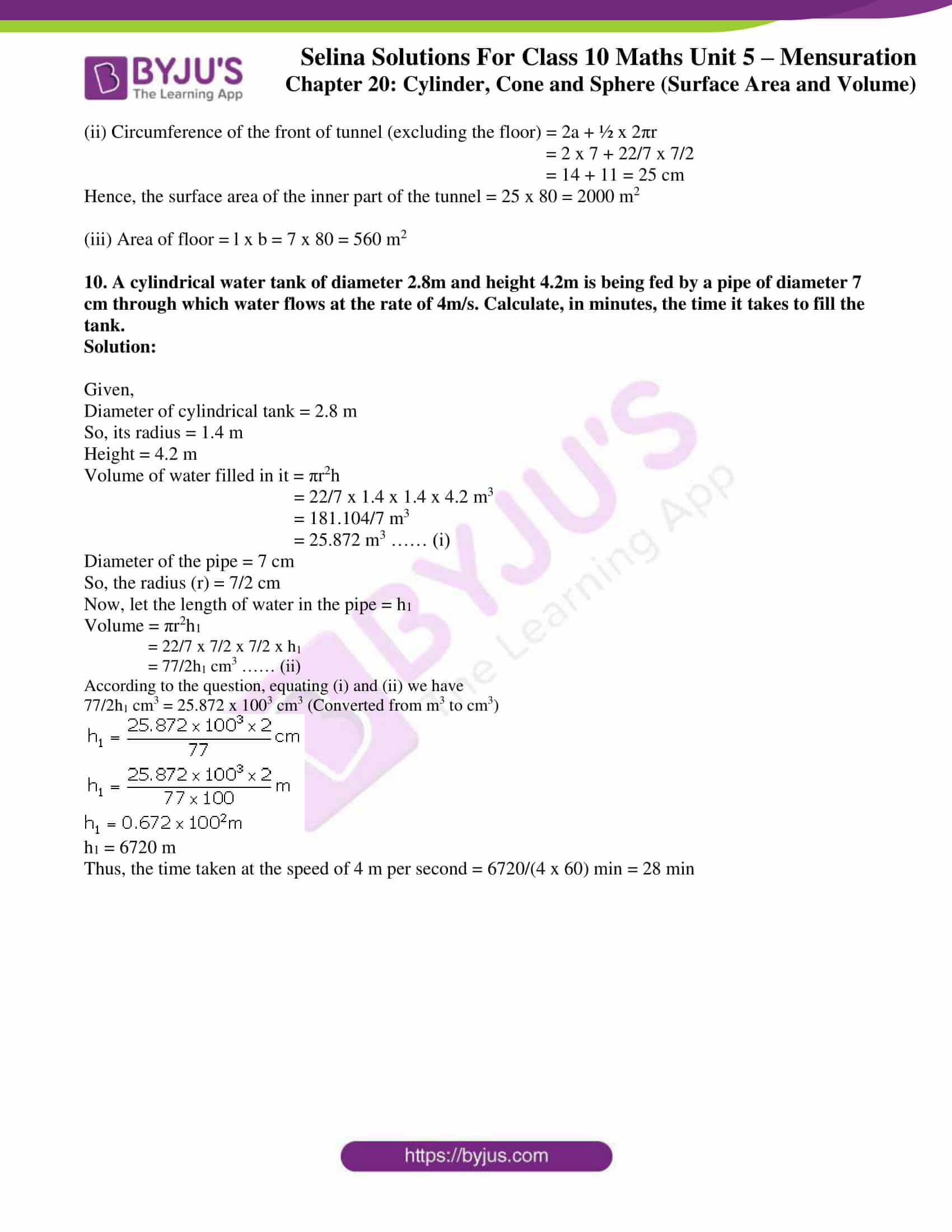### Access Selina Solutions Concise Maths Class 10 Chapter 20 Cylinder, Cone and Sphere (Surface Area and Volume) Exercise 20(G)

1. What is the least number of solid metallic spheres, each of 6 cm diameter, that should be melted and recast to form a solid metal cone whose height is 45 cm and diameter is 12 cm?

Solution:

Given,

Diameter of solid metallic sphere = 6 cm

So, its radius = 3 cm

Height of solid metal cone = 45

Diameter of metal cone = 12 cm

So, its radius = 6 cm

Now,

Let the number of solid metallic spheres be ‘n’

Volume of 1 sphere = 4/3 π (3)3

Volume of metallic cone = 1/3 π62 x 45

Hence, n = Volume of metal cone/ Volume of 1 sphere

n = (1/3 π62 x 45)/ (4/3 π (3)3)

= (6 x 6 x 45)/ (4 x 3 x 3 x 3)

n = 15

2. A largest sphere is to be carved out of a right circular cylinder of radius 7 cm and height 14 cm. Find the volume of the sphere. (Answer correct to the nearest integer)

Solution:

Radius of the largest sphere that can be formed inside the cylinder will be equal to the radius of the cylinder.

So, radius of the largest sphere = 7 cm

Volume of sphere = 4/3 π 73

= 4/3 x 22/7 x 7 x 7 x 7

= 4312/3

= 1437 cm3

3. A right circular cylinder having diameter 12 cm and height 15 cm is full of ice-cream. The ice-cream is to be filled in identical cones of height 12 cm and diameter 6 cm having a hemi-spherical shape on the top. Find the number of cones required.

Solution:

Given,

Diameter of the cylinder = 12 cm

So, its radius = 6 cm

Height of the cylinder = 15 cm

Diameter of the cone = 6 cm

So, its radius = 3 cm

Height of the cone = 12 cm

Radius of the hemisphere = 3 cm

Now,

Let the number of cones be ‘n’.

Volume of the cylinder = πr2h = π 62 x 15

Volume of an ice-cream cone with ice-cream = Volume of cone + Volume of hemisphere

= 1/3 π(3)2 x 12 + 2/3 π(3)2

= 36 π + 18 π

= 54 π cm3

Number of cones = Volume of cylinder/ Volume of ice-cream cone

= π 62 x 15 / 54 π

= (36 x 15)/ 54

= 10

Therefore, the number of cones required = 10

4. A solid is in the form of a cone standing on a hemisphere with both their radii being equal to 8 cm and the height of cone is equal to its radius. Find in terms of πthe volume of the solid.

Solution:

Given,

Radius of both cone and hemisphere = 8 cm

And, height = 8 cm

Hence,

Volume of the solid = Volume of cone + volume of hemisphere

= 1/3 πr2h + 2/3 πr3

= 1/3 π(8)2(8) + 2/3 π(8)3

= π(8)3

= 512 π cm3

5. The diameter of a sphere is 6 cm. It is melted and drawn into a wire of diameter 0.2 cm. Find the length of wire.

Solution:

Given,

Diameter of the sphere = 6 cm

So, its radius = 3 cm

And, volume = 4/3 πr3 = 4/3 x 22/7 x 3 x 3 x 3 = 792/7 cm3 ….. (i)

Diameter of cylindrical wire = 0.2 cm

So, the radius of the wire = 0.2/2 = 0.1 = 1/10 cm

Now, let length of wire = h

Volume = πr2 h = 22/7 x 1/10 x 1/10 x h cm3 = 22h/700 cm3 ….. (ii)

According to the question, equating (i) and (ii) we have

22h/700 = 792/7

h = 792/7 x 700/22

h = 3600 cm = 36 m

Therefore, length of the wire = 36 m

6. Determine the ratio of the volume of a cube to that of a sphere which will exactly fit inside the cube.

Solution:

Let edge of the cube = a

Then, volume of the cube = a x a x a = a3

The sphere that exactly fits in the cube will have radius ‘a/2’

So,

Volume of sphere = 4/3 πr3 = 4/3 x 22/7 x (a/2)3 = 4/3 x 22/7 x a3/8 = 11/21 a3

Now,

Volume of cube: Volume of sphere

= a3 : 11/21 a3

= 1 : 11/21

= 21 : 11

7. An iron pole consisting of a cylindrical portion 110 cm high and of base diameter 12 cm is surmounted by a cone 9 cm high. Find the mass of the pole, given that 1 cm3 of iron has 8 gm of mass (approx). (Take π = 355/113)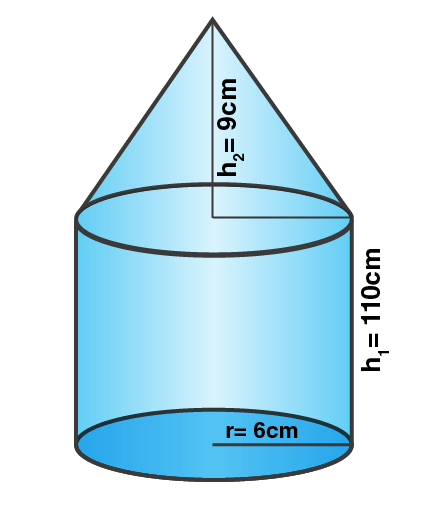Solution:

Given,

Radius of the base of poles (r) = 6 cm

Height of the cylindrical part (h1) = 110 cm

Height of the conical part (h2) = 9 cm

Now,

Total volume of the iron pole = πr2h1 + 1/3 πr2h2 = πr2 (h1 + 1/3h2)

= 355/113 x 6 x 6(110 + 1/3 x 9)

= 355/113 x 36 x 113

= 12780 cm3

Weight of 1 cm3 = 8 gm (Given)

Thus, the total weight = 12780 x 8 = 102240 gm = 102.24 kg

8. In the following diagram a rectangular platform with a semicircular end on one side is 22 meters long from one end to the other end. If the length of the half circumference is 11 meters, find the cost of constructing the platform, 1.5 meters high at the rate of Rs 4 per cubic meters.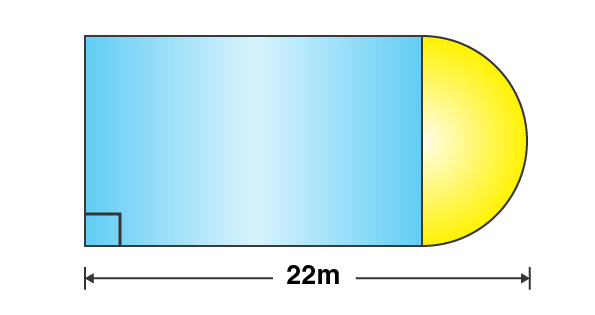Solution:

Given,

Length of the platform = 22 m

Circumference of semi-circle (c) = 11 m

So, its radius = (c x 2)/ (2 x π) = (11 x 7)/ 22 = 7/2 m

Thus, the breadth of the part = 7/2 x 2 = 7 m

And, length = 22 – 7/2 = 37/2 = 18.5 m

Now, area of platform = l x b + ½ πr2

= 18.5 x 7 + ½ x 22/7 x 7/2 x 7/2 m2

= 129.6 + 77/4 m2

= 148.75 m2

Also given, height of the platform = 1.5 m

So, the volume = 148.75 x 1.5 = 223.125 m3

Rate of construction = Rs 4 per m3

Therefore,

Total expenditure = Rs 4 x 223.125 = Rs 892.50

9. The cross-section of a tunnel is a square of side 7 m surmounted by a semicircle as shown in the following figure.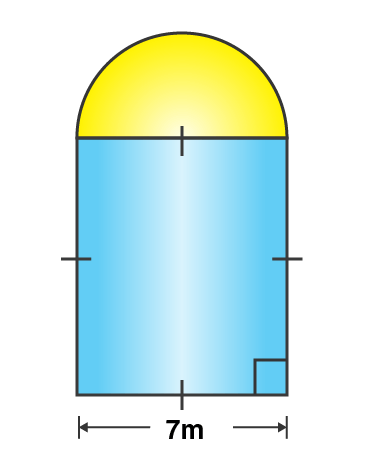The tunnel is 80 m long. Calculate:

(i) its volume

(ii) the surface area of the tunnel (excluding the floor) and

(iii) its floor area

Solution:

Given,

Side of square (a) = 7m

And, the radius of semi-circle = 7/2 m

Length of the tunnel = 80 m

Area of cross section of the front part = a2 + ½ πr2

= 7 x 7 + ½ x 22/7 x 7/2 x 7/2

= 49 + 77/4 m2

= (196 + 77)/ 4

= 273/4 m2

(i) Thus, the volume of the turnel = area of cross section x length of the turnel

= 273/4 x 80

= 5460 m3

(ii) Circumference of the front of tunnel (excluding the floor) = 2a + ½ x 2πr

= 2 x 7 + 22/7 x 7/2

= 14 + 11 = 25 cm

Hence, the surface area of the inner part of the tunnel = 25 x 80 = 2000 m2

(iii) Area of floor = l x b = 7 x 80 = 560 m2

10. A cylindrical water tank of diameter 2.8m and height 4.2m is being fed by a pipe of diameter 7 cm through which water flows at the rate of 4m/s. Calculate, in minutes, the time it takes to fill the tank.

Solution:

Given,

Diameter of cylindrical tank = 2.8 m

So, its radius = 1.4 m

Height = 4.2 m

Volume of water filled in it = πr2h

= 22/7 x 1.4 x 1.4 x 4.2 m3

= 181.104/7 m3

= 25.872 m3 …… (i)

Diameter of the pipe = 7 cm

So, the radius (r) = 7/2 cm

Now, let the length of water in the pipe = h1

Volume = πr2h1

= 22/7 x 7/2 x 7/2 x h1

= 77/2h1 cm3 …… (ii)

According to the question, equating (i) and (ii) we have

77/2h1 cm3 = 25.872 x 1003 cm3 (Converted from m3 to cm3)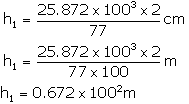h1 = 6720 m

Thus, the time taken at the speed of 4 m per second = 6720/(4 x 60) min = 28 min